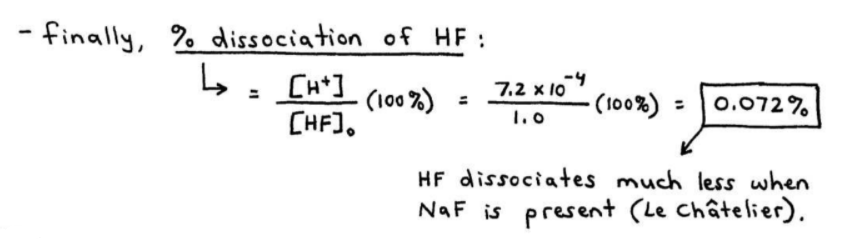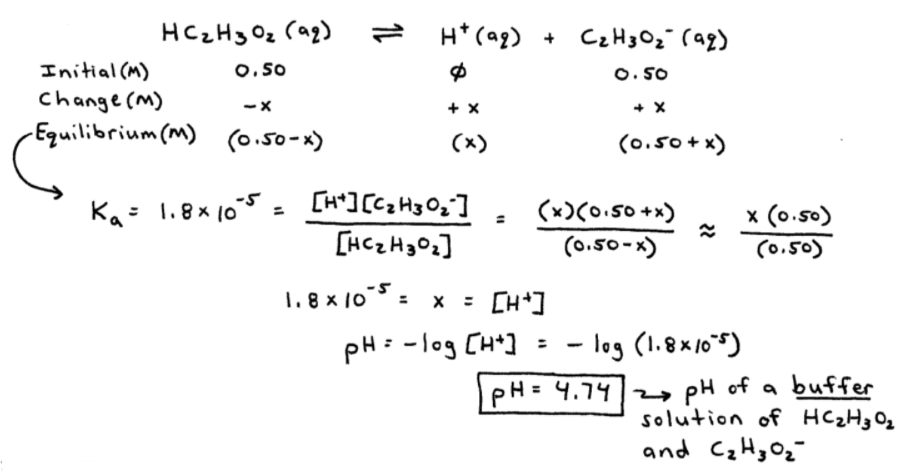# S15E1 - Buffer Solutions and the Common Ion Effect

## Buffers and the Common Ion Effect.

buffer is an aqueous solution containing a mixture of a weak acid and its conjugate base, or vice versa.

➞ Its pH changes very little when small amounts of strong acid or base are added.

➞ Alternate definition: solutions of acids or bases containing a "common ion."

Common Ion  =  an ion that is produced in common by both the acid or base, and the salt from which it comes from.

Buffered solutions are an example of Acid-Base Equilibria...

==========

## Acid-Base Equilibria

The solutions that we're talking about are solutions that, for example, contain not only the weak acid (HA), but also its salt (NaA, KA, etc...)

the addition of a common ion (A-) that's already a part of the acid's (HA) equilibrium expression, will cause a shift in equilibrium according to Le Chatelier's Principle.

this shift is called the "Common Ion Effect."

----------

ex:  In an experiment, it was found that the [H+]eq in a 1.0M HF solution was 2.7 x 10-2M, and the percent dissociation was 2.7%.

Now, calculate the [H+]eq and the % dissociation of HF in a solution containing (1.0M HF and 1.0M NaF).

The Ka for HF is 7.2 x 10-4.
_________

➞  1.  identify the major species first:  HF,  Na+ (neutral),  F-H2O (neutral)

➞  2.  so we have:Notice that the concentration of H+ at equilibrium is smaller than the previous [H+]eq of 2.7 x 10-2M.

This occurs because the additional [F-] from NaF shifts equilibrium to the left.

Away from  [H+]eq

➞  3.  finally, we can calcuate the percent dissociation of HF...

----------

### Calculate the Percent Dissociation of HF:As mentioned in the above image, HF dissociated much less in the presence of it's common ion (F-).

This is due to Le Chatelier's Principle.

==========

## Buffered Solutions

Here are 3 bulletpoint statements that we need to make about buffer solutions...

1. Buffer solutions occur with acid-base reactions containing a common ion.

2. A buffered solution is a solution that resists a change in its pH upon the addition of H+ or OH- ions.

3. Buffer problems in chemistry are nothing more than the weak acid (or base) solutions discussed in Section 14 ("Acids and Bases"), WITH the addition of a common ion, and they are solved using I.C.E. Tables.

----------

Let's do an example...

ex:  A buffer solution contains 0.50M acetic acid (Ka = 1.8 x 10-5) and 0.50M sodium acetate.

Calculate the pH of this solution.
_________

➞  1.  identify the major species first:  HC2H3O2,  Na+ (neutral),  C2H3O2-,  H2O (neutral)

➞  2.  so we have:Now, because this is a buffer solution, or "buffer," it resists a change in its pH upon the addition of acid (H+) or base (OH-).

==========

In my next post on this subject, namely Buffers and SECTION 15 - Applications of Acid-Base Equilibria,

We'll see how (and why) adding a very strong base (NaOH) to the [acetic acid / acetate] buffer solution will not cause the pH to increase very much from its 4.74 value.